Geometry 9-1 Translations
starstarstarstarstarstarstarstarstarstar
by Matthew Richardson
| 29 Questions
Note from the author:
A complete formative lesson with embedded slideshow, mini lecture screencasts, checks for understanding, practice items, mixed review, and reflection. I create these assignments to supplement each lesson of Pearson's Common Core Edition Algebra 1, Algebra 2, and Geometry courses. See also mathquest.net and twitter.com/mathquestEDU.
The outlined content above was added from outside of Formative.
The outlined content above was added from outside of Formative.
Solve It! Suppose you write the letters shown below on squares of tracing paper, so their shapes are visible from both sides.1
2
3
1
5 pts
Solve It! How can you move the squares from HUM to get ICE?
2
5 pts
Solve It! How can you move the squares from bob to get pod?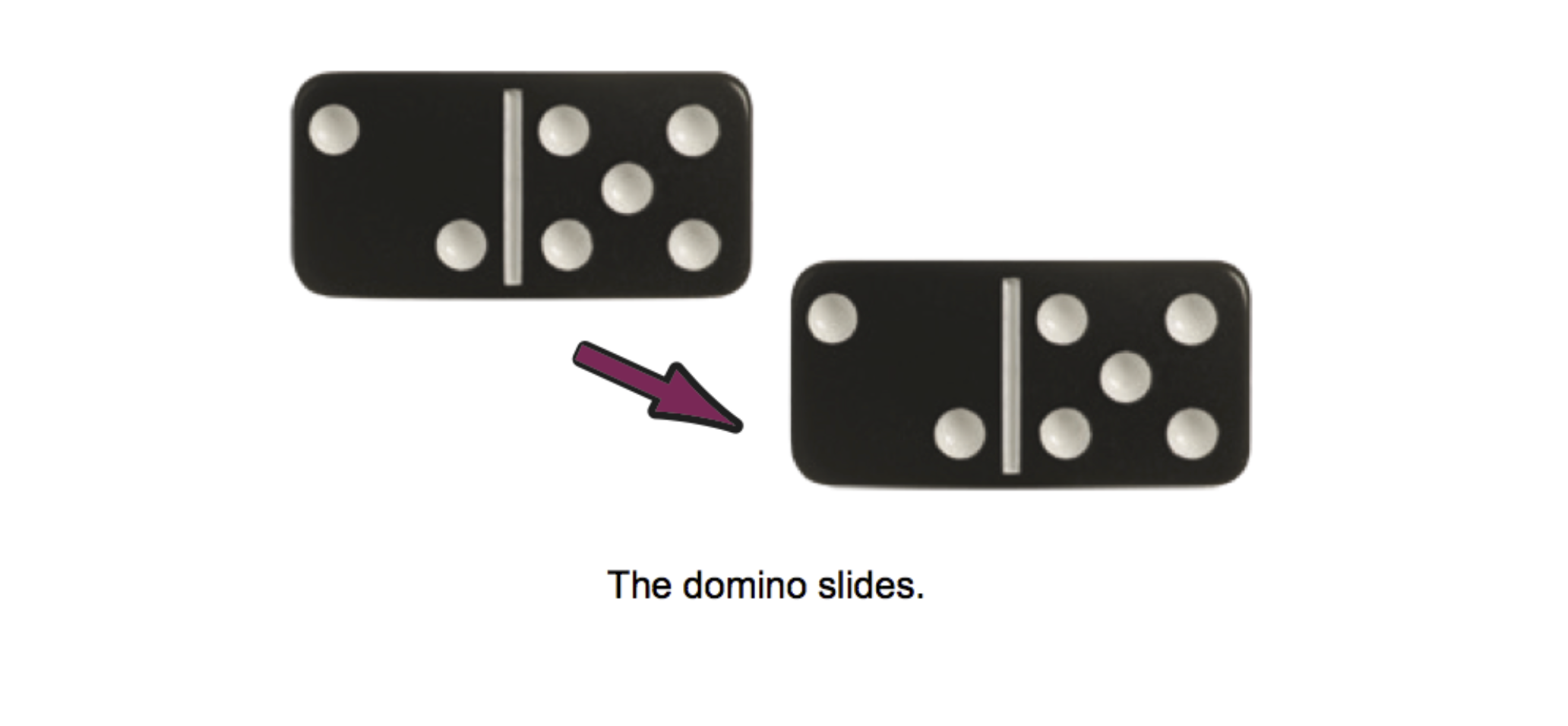4
10 pts
Vocabulary: Compare and contrast the geometric definition of transformation with its everyday English definition.
The outlined content above was added from outside of Formative.5
6
5
10 pts
Problem 1 Got It? Does the transformation appear to be a rigid motion? Explain.
Yes, the two figures appear to be congruent so the transformation seems to be a rigid motion.
Yes, the two figures are titled image and preimage so the transformation must be a rigid motion.
No, the two figures appear to be similar but not congruent so the transformation must not be a rigid motion.
6
10 pts
Problem 1 Got It? Does the transformation appear to be a rigid motion? Explain.
Yes, the two figures appear to be congruent so the transformation seems to be a rigid motion.
Yes, the two figures are titled image and preimage so the transformation must be a rigid motion.
No, the two figures appear to be similar but not congruent so the transformation must not be a rigid motion.The outlined content above was added from outside of Formative.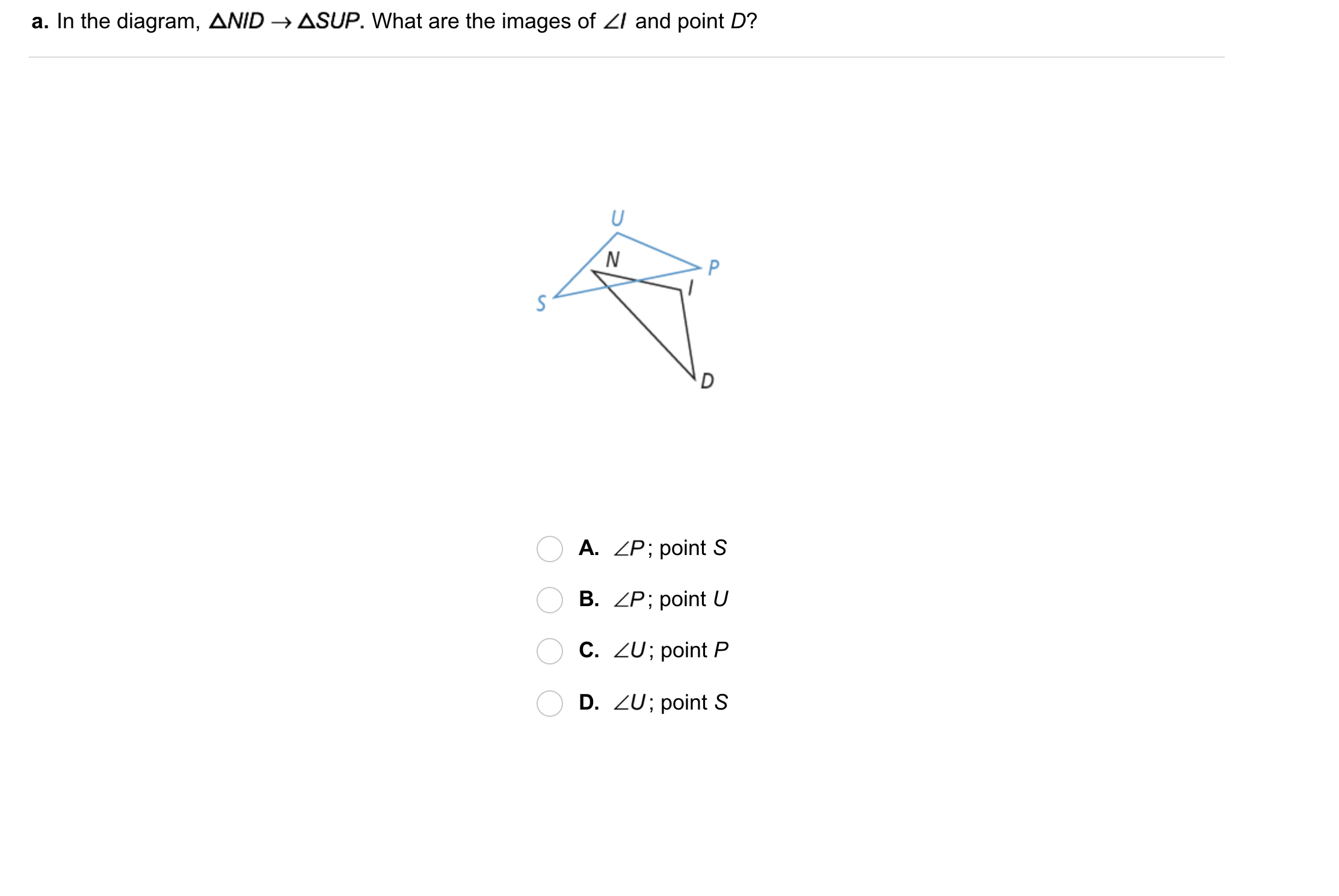7
7
10 pts
Problem 2 Got It?
A
B
C
D8
8
10 pts
Problem 2 Got It?
A
B
C
D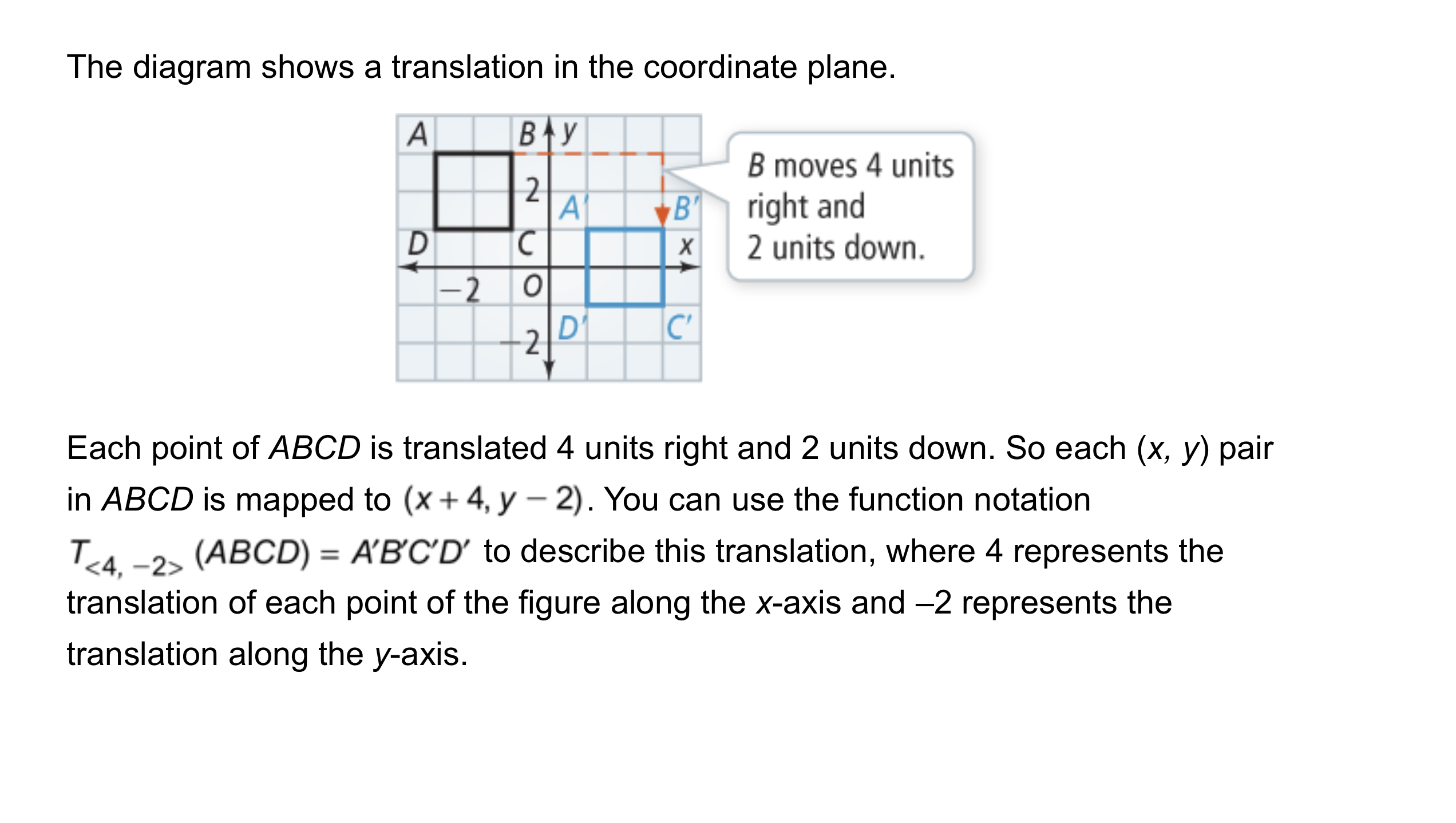The outlined content above was added from outside of Formative.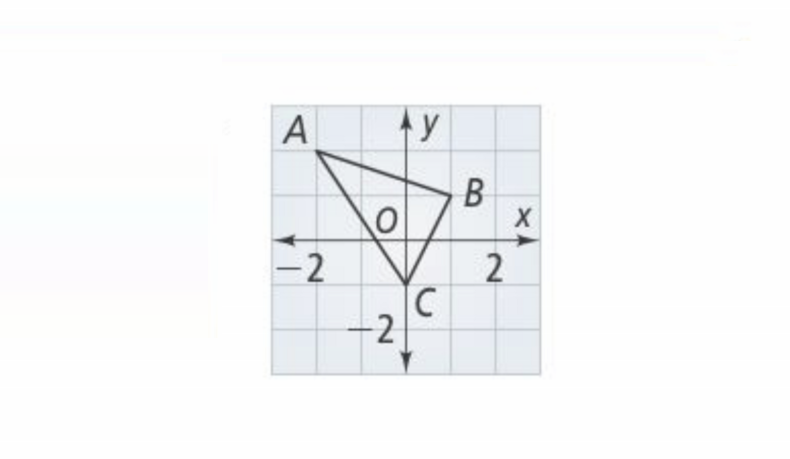9
10
11
9
10 pts
Problem 3 Got It? What are the vertices of the transformation?
Select all that apply.
A'(1, -4)
A'(-1, -2)
B'(2, -3)
B'(-1, -3)
C'(-5, 1)
C'(1, -5)
10
30 pts
Problem 3 Got It?
1. Copy △ABC onto the canvas in black.
2. Perform the translation below and graph the post-translation image in blue or green.
3. Draw these segments in red.
11
10 pts
Problem 3 Got It? Reasoning: Consider the segments you drew in step 3 of the previous item. What relationships exist among these three segments? Select all that apply.
Two of the segments are parallel, but the third has a different slope.
All three segments are congruent.
All three segments are parallel.
All of the segments have a slope of -4.
Two of the segments have a slope of 4.
The outlined content above was added from outside of Formative.12
12
10 pts
Problem 4 Got It?
A
B
C
D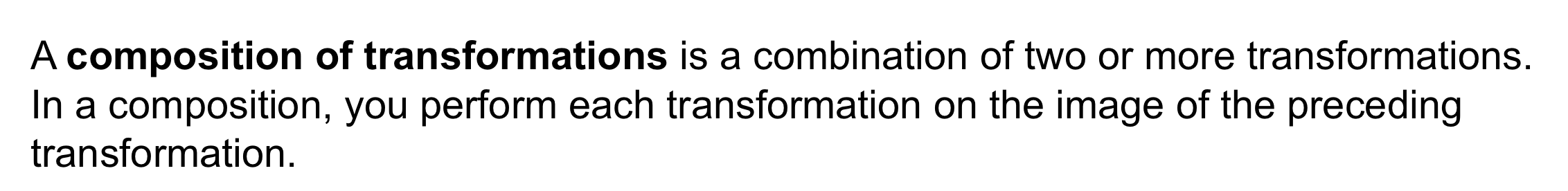The outlined content above was added from outside of Formative.13
13
10 pts
Problem 5 Got It?
A
B
C
D14
14
10 pts
A
B
C
D
15
20 pts
Graphing: Copy ▱NILE to the coordinate plane in black then graph its translation in blue or green.
Label vertices and include all relevant graph detail.16
16
10 pts
A
B
C
D17
10 pts
Vocabulary: What is true about a transformation that is not a rigid motion? Include a sketch of an example.18
18
10 pts
Error Analysis: Your friend says the transformation △ABC →△PQR is a translation. Explain and correct her error.
19
10 pts
Reasoning: Write the translation as composition of a horizontal translation and a vertical translation.20
10 pts
Review Lesson 8-1: Navigation: An airplane landed at a point 100 km east and 420 km south from where it took off. If the airplane flew in a straight line from where it took off to where it lands, how many kilometers did it fly? Enter only the number rounded to the nearest kilometer.
21
30 pts
Review Lesson 4-7: Proof: Complete the proof on the canvas.
22
10 pts
Review Lesson 3-8: The equations on the left go through a specific point A and are perpendicular to a specific line. Match each equation with its point A and equation of a perpendicular line on the right.
• x = -1
• y = -2
• y = -x + 1
• A(1, -2); x = -2
• A(-1, -1); y = 1
• A(-1, 2); y = x23
10 pts
Vocabulary Review: Fill in the blanks to complete the sentence:

If two triangles are congruent, corresponding angle measures are the same/different and corresponding side lengths are the same/different.
the same; the same
the same; different
different; the same
different: different24
25
26
27
24
5 pts
Respond with yes or no.
25
5 pts
Respond with yes or no.
26
5 pts
Right
Left
Up
Down
27
5 pts
Enter only a number to represent the distance.
28
10 pts
Key Concept: Translation: Complete each sentence with the correct word from the left column.
• some
• all
• change
• preserve
• Translations move __?__ points in a figure the same distance, in the same direction.
• Translations __?__ side lengths of the figure.
• Translations __?__ angle measures of the figure.
29
10 pts
Reflection: Math success